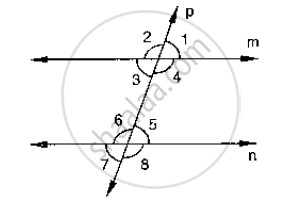# In the Below Fig, P is a Transversal to Lines M and N,∠2 = 120° and A∠5 = 60°. Prove that M || N. - Mathematics

In the below fig, p is a transversal to lines m and n,∠2 = 120° and a∠5 = 60°. Prove that m || n.#### Solution

Given that

∠2 = 120°, ∠5 = 60°

To prove

∠2 + ∠1 = 180°            [ ∵ Linear pair]

120° + ∠1 = 180°

∠1 = 180° -120°

∠1 = 60°

Since ∠1 = ∠5 = 60°

∴ m || n   [As pair of corresponding angles are equal]

Is there an error in this question or solution?
Chapter 10: Lines and Angles - Exercise 10.4 [Page 47]

#### APPEARS IN

RD Sharma Mathematics for Class 9
Chapter 10 Lines and Angles
Exercise 10.4 | Q 14 | Page 47

Share